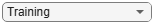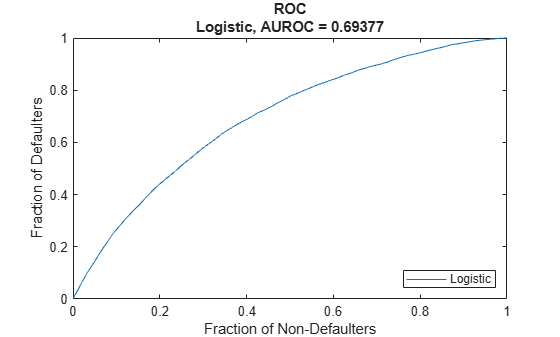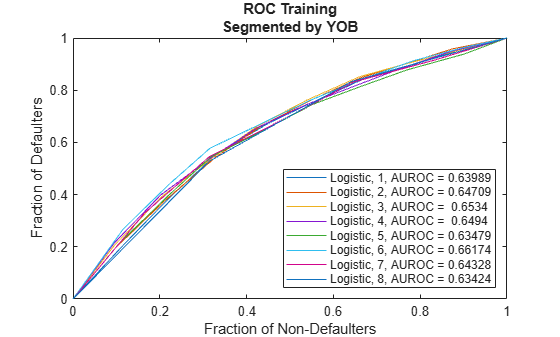# modelDiscrimination

Compute AUROC and ROC data

Since R2020b

## Syntax

``DiscMeasure = modelDiscrimination(pdModel,data)``
``[DiscMeasure,DiscData] = modelDiscrimination(___,Name,Value)``

## Description

example

````DiscMeasure = modelDiscrimination(pdModel,data)` computes the area under the receiver operating characteristic curve (AUROC). `modelDiscrimination` supports segmentation and comparison against a reference model.```

example

````[DiscMeasure,DiscData] = modelDiscrimination(___,Name,Value)` specifies options using one or more name-value pair arguments in addition to the input arguments in the previous syntax.```

## Examples

collapse all

This example shows how to use `fitLifetimePDModel` to fit data with a `Logistic` model and then generate the area under the receiver operating characteristic curve (AUROC) and ROC curve.

```load RetailCreditPanelData.mat disp(head(data))```
``` ID ScoreGroup YOB Default Year __ __________ ___ _______ ____ 1 Low Risk 1 0 1997 1 Low Risk 2 0 1998 1 Low Risk 3 0 1999 1 Low Risk 4 0 2000 1 Low Risk 5 0 2001 1 Low Risk 6 0 2002 1 Low Risk 7 0 2003 1 Low Risk 8 0 2004 ```
`disp(head(dataMacro))`
``` Year GDP Market ____ _____ ______ 1997 2.72 7.61 1998 3.57 26.24 1999 2.86 18.1 2000 2.43 3.19 2001 1.26 -10.51 2002 -0.59 -22.95 2003 0.63 2.78 2004 1.85 9.48 ```

Join the two data components into a single data set.

```data = join(data,dataMacro); disp(head(data))```
``` ID ScoreGroup YOB Default Year GDP Market __ __________ ___ _______ ____ _____ ______ 1 Low Risk 1 0 1997 2.72 7.61 1 Low Risk 2 0 1998 3.57 26.24 1 Low Risk 3 0 1999 2.86 18.1 1 Low Risk 4 0 2000 2.43 3.19 1 Low Risk 5 0 2001 1.26 -10.51 1 Low Risk 6 0 2002 -0.59 -22.95 1 Low Risk 7 0 2003 0.63 2.78 1 Low Risk 8 0 2004 1.85 9.48 ```

Partition Data

Separate the data into training and test partitions.

```nIDs = max(data.ID); uniqueIDs = unique(data.ID); rng('default'); % for reproducibility c = cvpartition(nIDs,'HoldOut',0.4); TrainIDInd = training(c); TestIDInd = test(c); TrainDataInd = ismember(data.ID,uniqueIDs(TrainIDInd)); TestDataInd = ismember(data.ID,uniqueIDs(TestIDInd));```

Create a `Logistic` Lifetime PD Model

Use `fitLifetimePDModel` to create a `Logistic` model.

```pdModel = fitLifetimePDModel(data(TrainDataInd,:),"Logistic",... 'AgeVar','YOB',... 'IDVar','ID',... 'LoanVars','ScoreGroup',... 'MacroVars',{'GDP','Market'},... 'ResponseVar','Default'); disp(pdModel)```
``` Logistic with properties: ModelID: "Logistic" Description: "" UnderlyingModel: [1x1 classreg.regr.CompactGeneralizedLinearModel] IDVar: "ID" AgeVar: "YOB" LoanVars: "ScoreGroup" MacroVars: ["GDP" "Market"] ResponseVar: "Default" WeightsVar: "" ```

Display the underlying model.

`pdModel.UnderlyingModel `
```ans = Compact generalized linear regression model: logit(Default) ~ 1 + ScoreGroup + YOB + GDP + Market Distribution = Binomial Estimated Coefficients: Estimate SE tStat pValue __________ _________ _______ ___________ (Intercept) -2.7422 0.10136 -27.054 3.408e-161 ScoreGroup_Medium Risk -0.68968 0.037286 -18.497 2.1894e-76 ScoreGroup_Low Risk -1.2587 0.045451 -27.693 8.4736e-169 YOB -0.30894 0.013587 -22.738 1.8738e-114 GDP -0.11111 0.039673 -2.8006 0.0051008 Market -0.0083659 0.0028358 -2.9502 0.0031761 388097 observations, 388091 error degrees of freedom Dispersion: 1 Chi^2-statistic vs. constant model: 1.85e+03, p-value = 0 ```
`pdModel.UnderlyingModel.Coefficients`
```ans=6×4 table Estimate SE tStat pValue __________ _________ _______ ___________ (Intercept) -2.7422 0.10136 -27.054 3.408e-161 ScoreGroup_Medium Risk -0.68968 0.037286 -18.497 2.1894e-76 ScoreGroup_Low Risk -1.2587 0.045451 -27.693 8.4736e-169 YOB -0.30894 0.013587 -22.738 1.8738e-114 GDP -0.11111 0.039673 -2.8006 0.0051008 Market -0.0083659 0.0028358 -2.9502 0.0031761 ```

Model Discrimination to Generate AUROC and ROC

Model "discrimination" measures how effectively a model ranks customers by risk. You can use the AUROC and ROC outputs to determine whether customers with higher predicted PDs actually have higher risk in the observed data.

```DataSetChoice ="Training"; if DataSetChoice=="Training" Ind = TrainDataInd; else Ind = TestDataInd; end DiscMeasure = modelDiscrimination(pdModel,data(TrainDataInd,:),'ShowDetails',true,'DataID',DataSetChoice); disp(DiscMeasure)```
``` AUROC Segment SegmentCount WeightedCount _______ __________ ____________ _____________ Logistic, Training 0.69377 "all_data" 3.881e+05 3.881e+05 ```

Visualize the ROC for the `Logistic` model using `modelDiscriminationPlot`.

`modelDiscriminationPlot(pdModel,data(TrainDataInd,:)); `Data can be segmented to get the AUROC per segment and the corresponding ROC data.

```SegmentVar ="YOB"; DiscMeasure = modelDiscrimination(pdModel,data(Ind,:),'ShowDetails',true,'SegmentBy',SegmentVar,'DataID',DataSetChoice); disp(DiscMeasure)```
``` AUROC Segment SegmentCount WeightedCount _______ _______ ____________ _____________ Logistic, YOB=1, Training 0.63989 1 58092 58092 Logistic, YOB=2, Training 0.64709 2 56723 56723 Logistic, YOB=3, Training 0.6534 3 55524 55524 Logistic, YOB=4, Training 0.6494 4 54650 54650 Logistic, YOB=5, Training 0.63479 5 53770 53770 Logistic, YOB=6, Training 0.66174 6 53186 53186 Logistic, YOB=7, Training 0.64328 7 36959 36959 Logistic, YOB=8, Training 0.63424 8 19193 19193 ```

Visualize the ROC segmented by `YOB`, `ScoreGroup`, or `Yea`r using `modelDiscriminationPlot`.

`modelDiscriminationPlot(pdModel,data(Ind,:),'SegmentBy',SegmentVar,'DataID',DataSetChoice);`## Input Arguments

collapse all

Probability of default model, specified as a `Logistic`, `Probit`, or `Cox` object previously created using `fitLifetimePDModel`. Alternatively, you can create a custom probability of default model using `customLifetimePDModel`.

Note

The `'ModelID'` property of the `pdModel` object is used as the identifier or tag for `pdModel`.

Data Types: `object`

Data, specified as a `NumRows`-by-`NumCols` table with projected predictor values to make lifetime predictions. The predictor names and data types must be consistent with the underlying model.

Data Types: `table`

### Name-Value Arguments

Specify optional pairs of arguments as `Name1=Value1,...,NameN=ValueN`, where `Name` is the argument name and `Value` is the corresponding value. Name-value arguments must appear after other arguments, but the order of the pairs does not matter.

Before R2021a, use commas to separate each name and value, and enclose `Name` in quotes.

Example: ```[PerfMeasure,PerfData] = modelDiscrimination(pdModel,data(Ind,:),'DataID',"DataSetChoice")```

Data set identifier, specified as the comma-separated pair consisting of `'DataID'` and a character vector or string.

Data Types: `char` | `string`

Name of a column in the data input, not necessarily a model variable, to be used to segment the data set, specified as the comma-separated pair consisting of `'SegmentBy'` and a character vector or string.

One AUROC value is reported for each segment and the corresponding ROC data for each segment is returned in the `PerfData` optional output.

Data Types: `char` | `string`

Since R2022a

Indicates if the output includes columns showing segment value, segment count, and weighted count, specified as the comma-separated pair consisting of `'ShowDetails'` and a scalar logical.

Data Types: `logical`

Conditional PD values predicted for `data` by the reference model, specified as the comma-separated pair consisting of `'ReferencePD'` and a `NumRows`-by-`1` numeric vector. The `modelDiscrimination` output information is reported for both the `pdModel` object and the reference model.

Data Types: `double`

Identifier for the reference model, specified as the comma-separated pair consisting of `'ReferenceID'` and a character vector or string. `'ReferenceID'` is used in the `modelDiscrimination` output for reporting purposes.

Data Types: `char` | `string`

## Output Arguments

collapse all

AUROC information for each model and each segment., returned as a table. `DiscMeasure` has a single column named `'AUROC'` and the number of rows depends on the number of segments and whether you use a `ReferenceID` for a reference model and `ReferencePD` for reference data. The row names of `DiscMeasure` report the model IDs, segment, and data ID. If the optional `ShowDetails` name-value argument is `true`, the `DiscMeasure` output displays `Segment`, `SegmentCount`, and `WeightedCount` columns.

Note

If you do not specify `SegmentBy` and use `ShowDetails` to request the segment details, the two columns are added and show the `Segment` column as `"all_data"` and the sample size (minus missing values) for the `SegmentCount` column.

ROC data for each model and each segment, returned as a table. There are three columns for the ROC data, with column names `'X'`, `'Y'`, and `'T'`, where the first two are the X and Y coordinates of the ROC curve, and T contains the corresponding thresholds.

If you use `SegmentBy`, the function stacks the ROC data for all segments and `DiscData` has a column with the segmentation values to indicate where each segment starts and ends.

If reference model data is given using `ReferenceID` and `ReferencePD`, the `DiscData` outputs for the main and reference models are stacked, with an extra column `'ModelID'` indicating where each model starts and ends.

collapse all

### Model Discrimination

Model discrimination measures the risk ranking.

Higher-risk loans should get higher predicted probability of default (PD) than lower-risk loans. The `modelDiscrimination` function computes the Area Under the Receiver Operator Characteristic curve (AUROC), sometimes called simply the Area Under the Curve (AUC). This metric is between 0 and 1 and higher values indicate better discrimination.